Next: Goals of Competitive Learning Up: Some Competitive Learning Methods Previous: Introduction

# Common Properties and Notational Conventions

The models described in this report share several architectural properties which are described in this chapter. For simplicity, we will refer to any of these models as network even if the model does not belong to what is usually understood as ``neural network''.

Each network consists of a set of N units:Each unit c has an associated reference vectorindicating its position or receptive field center in input space.

Between the units of the network there exists a (possibly empty) setof neighborhood connections which are unweighted and symmetric: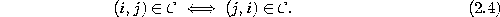These connections have nothing to do with the weighted connections found, e.g., in multi-layer perceptrons (Rumelhart et al., 1986). They are used in some methods to extend the adaptation of the winner (see below) to some of its topological neighbors.

For a unit c we denote withthe set of its direct topological neighbors:The n-dimensional input signals are assumed to be generated either according to a continuous probability density function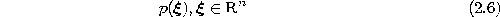or from a finite training data set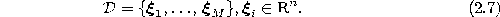For a given input signalthe winneramong the units inis defined as the unit with the nearest reference vectorwhereby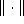denotes the Euclidean vector norm. In case of a tie among several units one of them is chosen to be the winner by throwing a fair dice. In some cases we will denote the current winner simply by s (omitting the dependency on). If not only the winner but also the second-nearest unit or even more distant units are of interest, we denote with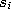the i-nearest unit (is the winner,is the second-nearest unit, etc.).

Two fundamental and closely related concepts from computational geometry are important to understand in this context. These are the Voronoi Tessellation and the Delaunay Triangulation:

Given a set of vectorsin(see figure 2.1 a), the Voronoi Regionof a particular vector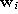is defined as the set of all points infor whichis the nearest vector: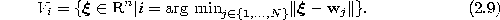In order for each data point to be associated to exactly one Voronoi region we define (as previously done for the winner) that in case of a tie the corresponding point is mapped at random to one of the nearest reference vectors. Alternatively, one could postulate general positions for all data points and reference vectors in which case a tie would have zero probability.

It is known, that each Voronoi regionis a convex area, i.e.The partition offormed by all Voronoi polygons is called Voronoi Tessellation or Dirichlet Tessellation (see figure 2.1 b). Efficient algorithms to compute it are only known for two-dimensional data sets (Preparata and Shamos, 1990). The concept itself, however, is applicable to spaces of arbitrarily high dimensions.

If one connects all pairs of points for which the respective Voronoi regions share an edge (an (n-1)-dimensional hyperface for spaces of dimension n) one gets the Delaunay Triangulation (see figure 2.1 c). This triangulation is special among all possible triangulation in various respects. It is, e.g., the only triangulation in which the circumcircle of each triangle contains no other point from the original point set than the vertices of this triangle. Moreover, the Delaunay triangulation has been shown to be optimal for function interpolation (Omohundro, 1990). The competitive Hebbian learning method generates a subgraph of the Delaunay triangulation which is limited to those areas of the input space where data is found.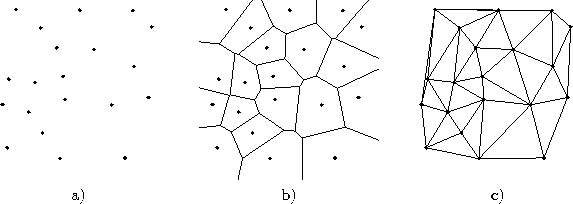Figure 2.1:  a) Point set in, b) corresponding Voronoi tessellation, c) corresponding Delaunay triangulation.

For convenience we define the Voronoi Region of a unit, as the Voronoi region of its reference vector: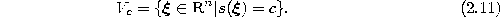In the case of a finite input data setwe denote for a unit c with the term Voronoi Set the subsetoffor which c is the winner (see figure 2.2):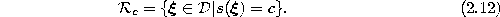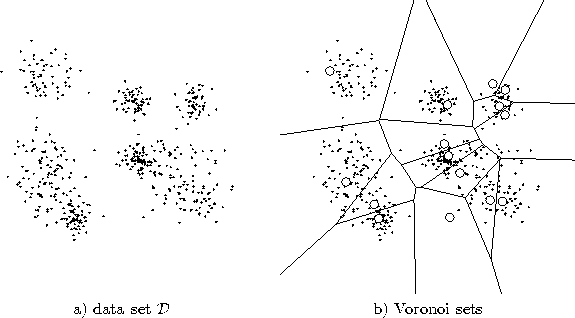Figure 2.2:   An input data setis shown (a) and the partition of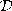into Voronoi sets for a particular set of reference vectors (b). Each Voronoi set contains the data points within the corresponding Voronoi field.Next: Goals of Competitive Learning Up: Some Competitive Learning Methods Previous: Introduction

Bernd Fritzke
Sat Apr 5 18:17:58 MET DST 1997